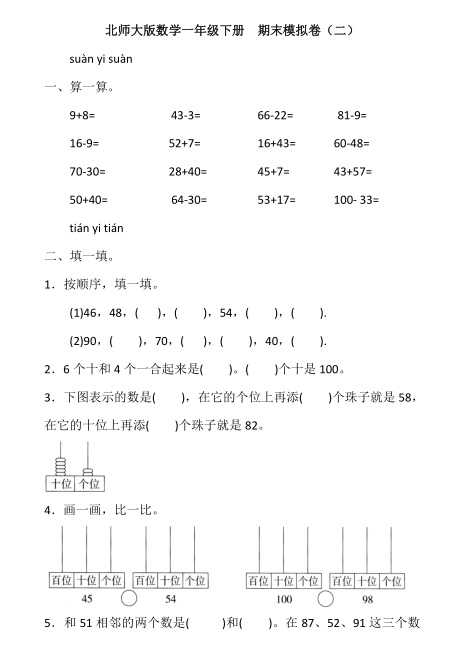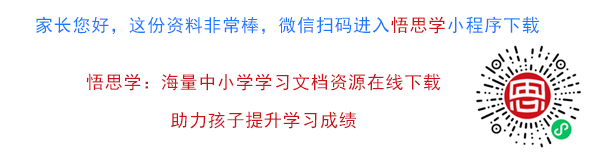suàn yi suàn

9+8= 43-3= 66-22= 81-9=
16-9= 52+7= 16+43= 60-48=
70-30= 28+40= 45+7= 43+57=

【PDF文档7页】北师大版数学一年级下册期末模拟卷（二）（含答案） A4电子版资料_可直接打印_会员免费下载##### 评论信息